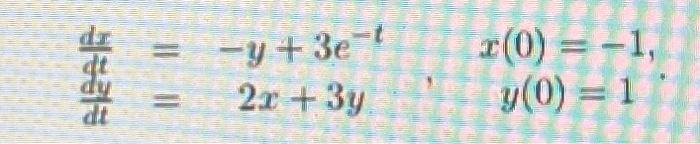# (Solved): solve the ivps by sub or elimination dtdx=y+3etdtdy=2x+3y,x(0)=1,y(0)=1 ...

solve the ivps by sub or eliminationWe have an Answer from Expert

Put d/dt=D

Multiple equation (1 by 3 and subtract (1 and (2

Operate equation (1

We have an Answer from Expert